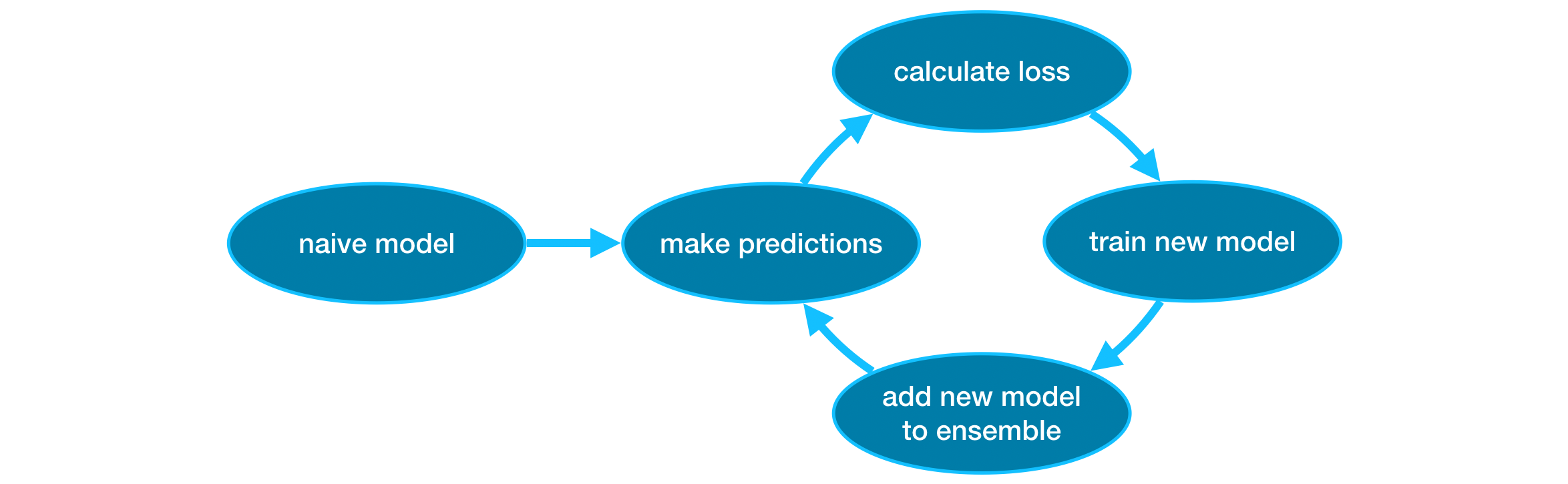# Kaggle Challenge 14 - XGBoost

ljsk99499·2021년 8월 16일
0

## Kaggle Challenge August 2nd~

목록 보기
11/12#### Introduction

For much of this course, you have made predictions with the random forest method, which achieves better performance than a single decision tree simply by averaging the predictions of many decision trees.
We refer to the random forest method as an "ensemble method". By definition, ensemble methods combine the predictions of several models (e.g., several trees, in the case of random forests).### Example

We begin by loading the training and validation data in X_train, X_valid, y_train, and y_valid.

from xgboost import XGBRegressor

my_model = XGBRegressor()
my_model.fit(X_train, y_train)
from sklearn.metrics import mean_absolute_error

predictions = my_model.predict(X_valid)
print("Mean Absolute Error: " + str(mean_absolute_error(predictions, y_valid)))

Mean Absolute Error: 238794.73582819404

### Parameter Tuning

XGBoost has a few parameters that can dramatically affect accuracy and training speed. The first parameters you should understand are:

my_model = XGBRegressor(n_estimators=500)
my_model.fit(X_train, y_train)
my_model = XGBRegressor(n_estimators=500)
my_model.fit(X_train, y_train,
early_stopping_rounds=5,
eval_set=[(X_valid, y_valid)],
verbose=False)
my_model = XGBRegressor(n_estimators=1000, learning_rate=0.05)
my_model.fit(X_train, y_train,
early_stopping_rounds=5,
eval_set=[(X_valid, y_valid)],
verbose=False)
my_model = XGBRegressor(n_estimators=1000, learning_rate=0.05, n_jobs=4)
my_model.fit(X_train, y_train,
early_stopping_rounds=5,
eval_set=[(X_valid, y_valid)],
verbose=False)